Linear Regression

Linear Regression is one of the popular algorithms in Machine Learning and perhaps in the statistic community as well. In this post, I will dive you into the math behind linear regression and how it actually works. I hope you are aware of equations, not any high-level linear algebra or statistics, and a little bit of Machine Learning also.

1. Introduction
2. Intuition
3. Why is the name Linear Regression?
4. Assumptions in Linear Regression
5. Model Representation
6. Loss function in Linear Regression
8. Overfitting and Underfitting
9. Regularization
11. Case Study on Boston House Prediction Dataset

1. Introduction

Linear Regression is studied to understand the relation between the output variable(y) and the input variables(X). If there is only one input variable then we called it Simple Linear Regression and for more than one input variable, it is referred to as Multiple Linear Regression.

A Machine Learning regression problem can be viewed as finding the real value of the output variable(y) given input features(X). So the model is trained to find out the relation between output and input. The model will try to learn the statistical relationship between the variables, not the deterministic relationship. In the deterministic relationship, one variable can be perfectly expressed as others like converting student marks into percentages given CGPA. In the case of a statistical relationship, one variable cannot be perfectly expressed as another. For example, determining the relation between the price of the flat and area of flat.

2. Intuition

Before we go into the mathematical background of Linear regression let’s try to build the intuition with the help of real-world problems.  Let’s say we want to predict the age of the person based on the weight and we have the following data with us.If we plot the above 2-D data then it will look similar to the following:The whole aim of the Linear Regression is to find the best line (In 2-D) through all the points that can minimize the error. The best-fitting line is called a regression line. In higher dimensional data we called it a regression plane.

Now If I ask you which of the above is the best line through all the points?

One can intuitively say that the line in figure 2 is the best line through all the points. As you can see the red line is much closer to all the points than the remaining 2 lines in other figures.

I hope now you have a high-level idea of the Linear Regression model and what it actually does. In the next, I will go step by step into a little bit of maths.

3. Why is the name Linear Regression?

The linear regression was found a lot of years ago and is studied by different researchers in different ways. The name linear is there because it is borrowed from the linear model. A linear model is one that assumes the linear relationship between the output variable(y) and input features(X). The term regression is because it solves the regression problem in ML.

This is how the name is given Linear Regression. More generally we can say that y(output) is calculated from the linear combination of X(inputs).

4. Assumptions in Linear Regression

In order to understand how to use linear regression in practice, we must understand the assumptions of it. The assumptions are as follows:

4.1 Homoscedasticity:

It describes a condition in which the error term (which we want to minimize by the model) is similar across all the values of independent variables X. If there is no specific pattern observed in the data then we called it heteroscedasticity. The most convenient way to check whether data is homoscedastic or heteroscedastic is to plot the scatterplot between the residual values and the predicted values.

4.2 Linear relationship:

By this assumption, there is a linear relationship between the output variable(y) and input features(X). This can be validated by using the scatterplot between the features and output.

4.3 No Multicollinearity:

Multicollinearity occurs in the data when the input features are highly interrelated with each other. The heatmap is used to determine the correlation between each feature. This value lies between 0 and 1. 0 indicates that the particular two features are not correlated to each other and exactly the opposite goes for 1.

4.4 Multivariate normal distribution:

The error produced by the model while predicting the values follows the normal distribution.

4.5 Autocorrelation between the errors:

This assumption is mostly applicable to the time series data. When the current value of the residual is dependent on its previous value then we can say that there exists the autocorrelation between the residuals.

However, now we have seen most of the assumptions of the linear regression that one has to keep in mind while building the regression model. These assumptions make sure that the linear model gives us the best possible result.

5. Model Representation:

Let’s try to recall the example that we have discussed in section 2 of predicting the age given the weight of the person. The equation of the straight line can be represented as follows:

y=mx + c

Where y = Output variable

m = slope of the line

X = input feature and

C = Intercept

In Machine Learning we rewrite the above equation as follows:

y^ = W1X1 + W0

Where y^ = Predicted output

Xi = input

W0, W1 = Parameters of the model

The above equation we saw is only when we have one input variable and one target variable. But the real world has more than 2 dimensions. So our changes to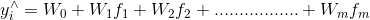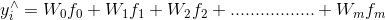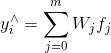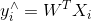Where fi = input features and 1<= i < m

f0 = 1

Wi = Parameters of the model

m = dimensions of the data

We can think of different values of Wi as the weight of each feature or influence of the different features on the output. If for a particular feature the W = 0 means that feature doesn’t have any effect on the output produced. Therefore it indirectly removes the influence of that particular input on the model.

6. Loss function in Linear Regression

The different values of the weights (W0, W1, ………, Wm) give us a different line in 2-D and different hyperplanes in m-dimensions. But how did the model come to know which line or hyperplane is the best fit for the data? Even how the model compares 2 different lines or hyperplanes.

Because of this reason, we introduce a loss function called Squared Loss. The mean squared loss defines how close yi to actual point ytrue.

For each point, the data we define a loss a follows: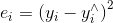Where yi^ =  Predicted value

yi = Actual value

By squaring we are getting rid of the negative values in case there is any.

We sum over all the errors produced by the model for all the points and take the values of the weights that line which minimizes the error. The mathematical equation for it can be represented as follows: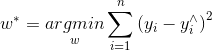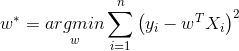This is also called an Ordinary Least Square Method.

Gradient descent is an optimization algorithm used for optimizing the parameters in order to reduce the given loss function.

In the case of linear regression, it does by adjusting the weights of the features. Firstly it randomly initializes the weights then it adjusts weights in such a way that the squared error is minimum. The squared loss is calculated for each pair of input and output data points. A learning rate (alpha) is used to determine the step size on each iteration throughout the procedure.

I have another blog that explains the GD in more detail. You can refer to it by the below link for more information (link here).

8. Overfitting and Underfitting

Overfitting is the condition when the model learns each and every small detail of training data which impacts the performance of the model on the completely unseen data.

Underfitting is a state in which the model learns nothing is not generalized well for the unseen data. The underfitting for the regression model can be simply the mean of the target variables.

The below 2 figures gives a clear idea of underfitting and overfitting in the linear regression model.How to find the best model?

The answer is depending on the model at hand we try to fit the model between overfitting and underfitting often called a good fit. As you might know, the aim of the whole Machine Learning model is to develop a generalized model that lies between high bias and high variance. Finding whether a model suffers from high bias or high variance is another responsibility of the developer.

10. Regularization

Regularization is the technique of penalizing the magnitude of the coefficient of the model. In regularization, we keep all the features the same number of features but the magnitude of the coefficient of it.

But how can we reduce the magnitude of the coefficient? For this purpose, we have a different regression that uses regularization to overcome this. They are Ridge regression, Lasso regression, and elastic net regression. I have covered all this technique in a different blog post. For more information, you can refer to Logistic Regression, Ridge regression, Lasso regression, and Elastic Net regression.

12. Conclusion

In this post, we have learned the concepts of linear regression as well as the maths behind it. Also, we have applied linear regression on real-life datasets. So I hope you are now able to relate the concepts that we have learned with the case study.

Thanks for reading! If you liked the blog then let me know your thoughts in the comment section.

Share

Lasso Regression

In this tutorial, I will brief you about the linear regression and the bias-variance problem